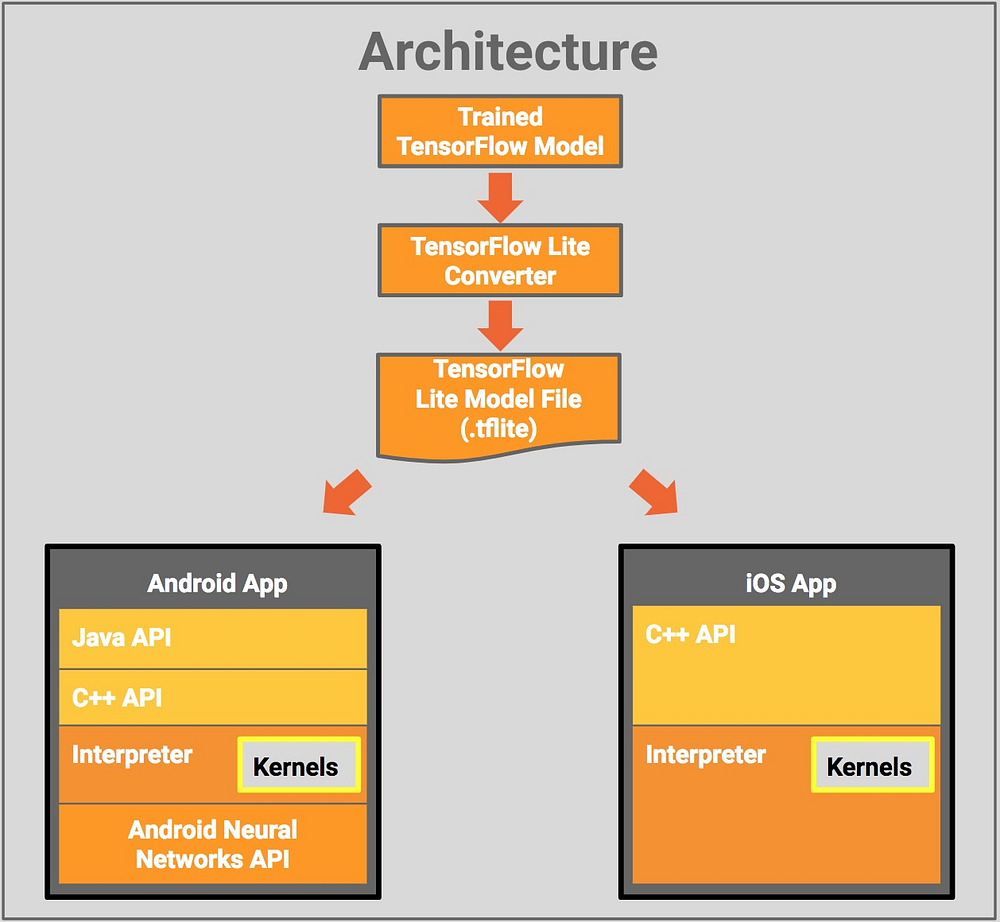# Deploy MobileNet model to Android Platform

Source: Deep Learning on Medium# Deploy MobileNet model to Android Platform

Hello again,

This blog is a continuation of the previous blog name “Memes Detection Android App using Deep Learning”. In this blog, we are going to deploy the model on an android environment.

Please go through my previous blog to understand the business problems and machine learning formulation for this problem.

# Part 2

In the previous blog, we build VGG19 model here we are going to fine-tune a pre-trained MobileNet Keras model.

What we are going to learn in this blog?

• Convert Keras model to TFLite model
• Deploy the model on an android platform

Below is a code snippet to fine-tune MobileNet Model.

`keras.backend.clear_session()IMG_SIZE = 224IMG_SHAPE = (IMG_SIZE, IMG_SIZE, 3)mobilenet_v1 = keras.applications.mobilenet.MobileNet(include_top=False, input_shape=IMG_SHAPE, )prediction_layer = keras.layers.Dense(2, activation='softmax')flatter = keras.layers.Flatten()model = keras.Sequential([ mobilenet_v1, flatter, prediction_layer])for layer in model2.layers: print(layer, layer.trainable)model.summary()model.compile(loss='binary_crossentropy',optimizer=keras.optimizers.Adamax(0.0005), metrics=['accuracy'])earliStop = EarlyStopping(monitor='val_loss', mode='min', verbose=1, patience=2)history = model.fit_generator( train_batch, steps_per_epoch=20, epochs=13, validation_data=validate_batch, callbacks=[earliStop], validation_steps=10,)`

# Result

Training LogLoss = 0.13648
Training Accuracy = 96.644 %

Validation LogLoss = 0.18804
Validation Accuracy = 95.446 %

Test LogLoss = 0.22778
Test Accuracy = 95.323 %

## Convert Keras model to TFLite model

`converter=tf.lite.TFLiteConverter.from_keras_model(model)tflite_model = converter.convert()`

then we can save the model using below code.

`open("/content/drive/My Drive/mobilenet/best_model3.tflite", "wb").write(tflite_model)`

Note: We have stored the model using .tflite file extension.

If you know the basics knowledge of android development then deploying “TF-Lite” model is trivial.

We have to appreciate this google codelab code snippet.

## Deploy the model on an android platform

The below step will helpful for you.

• After converting Keras model to TFlite model as shown above, save file as .tflite file extension.
• Create a simple android project, using Android Studio.
• In android project directory, create a folder called ‘Assets’.
• Store “model.tflite” file into that “Assets” folder.
• Create a “class_labels.txt” file in which output categories file. In our case, it should contain two class labels ie “Meme” and “Not Meme”. If you are working with 1000 classification problem then “class_labels.txt” will contain 1000 class labels.
• In google codelab project there is ‘ImageClassifier.Java’ file. For reference. It is an interpreter in google codelab case. Interpreter talks between .tflite model and android /java/ c++ client.

`android {.......aaptOptions { noCompress "tflite" noCompress "lite" }}dependencies {implementation 'org.tensorflow:tensorflow-lite:+'}`

final and last step, just added interpreter class.

Let’s understand our Interpreter class. Our interpreter class name is MemesClassifier.java, which can be found here.

`private static final String MODEL_PATH = "model.tflite";private static final String LABEL_PATH = "labels.txt";`

`## Load .tflite model/** * Memory-map the model file in Assets. */ private MappedByteBuffer loadModelFile(Context activity) throws IOException { AssetFileDescriptor fileDescriptor = activity.getAssets().openFd(MODEL_PATH); FileInputStream inputStream = new FileInputStream(fileDescriptor.getFileDescriptor()); FileChannel fileChannel = inputStream.getChannel(); long startOffset = fileDescriptor.getStartOffset(); long declaredLength = fileDescriptor.getDeclaredLength(); return fileChannel.map(FileChannel.MapMode.READ_ONLY, startOffset, declaredLength); }`

`/** * Reads label list from Assets. */private List<String> loadLabelList(Context activity) throws IOException { List<String> labelList = new ArrayList<String>(); BufferedReader reader = new BufferedReader(new InputStreamReader(activity.getAssets().open(LABEL_PATH))); String line; while ((line = reader.readLine()) != null) { labelList.add(line); } reader.close(); return labelList;}`

below method classify an image.

`/** * Classifies a frame from the preview stream. */public String classifyImages(Bitmap bitmap) { if (tflite == null) { Log.e(TAG, "Image classifier has not been initialized; Skipped."); return "Uninitialized Classifier."; } convertBitmapToByteBuffer(bitmap);// Here's where the magic happens!!!long startTime = SystemClock.uptimeMillis(); tflite.run(imgData, labelProbArray); long endTime = SystemClock.uptimeMillis(); Log.d(TAG, "Timecost to run model inference: " + Long.toString(endTime - startTime)); float findProba0 = labelProbArray; float findProba1 = labelProbArray; String result; if (findProba1 < findProba0) { result = IMAGE_STATUS_MEME; } else { result = IMAGE_STATUS_NOT_MEME; } return result;}`
• convertBitmapToByteBuffer: this method is used to convert image to array.

THAT’S IT.

Run the android studio project and supply test images to “classifyFrame” method. It will return whether an image is a Meme or Not a Meme.

Source code can be found here.

Also, don’t miss deploying Keras model to the flask app.

# Output

Android App Landing Page